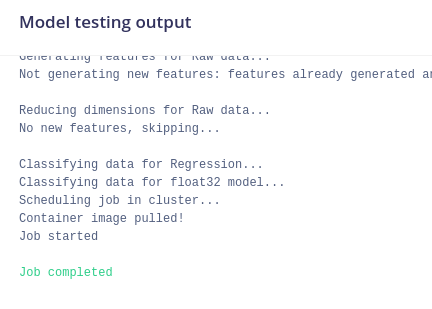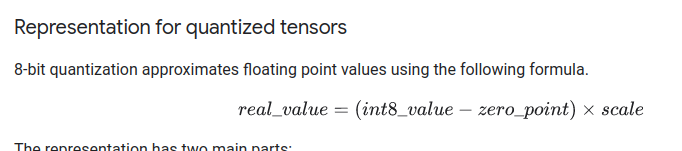# Model testing: model selection (float32 - int 8) (extra info)

Hello EI-Team,

If you perform model testing, currently, the float32 model is selected to classify the data.
Can I choose the int8 model?Regards,
Joeri

Hello,

Some extra info:

I aim to obtain a data frame with columns = [‘result’, ‘expected result’] for both float and int8 models.
Given this data frame, I can perform some analysis and make plots (for ex., Bland Altman plot), …

Note: I am working on a regression model.

Currently, I am downloading the results (Sample name, Expected outcome and Result) for the float32 model using the EI-API. The idea is to do this also for the int8 model.

However, I am working on an alternative, where I download both the float and int8 model files and perform inference on my local machine. Local, I also have the test data in JSON format as defined by Data acquisition format

So I downloaded the model and run a script; below is part of the code:

``````# load tflite model
interpreter = tf.lite.Interpreter(model_dir)

# get input and output tensors
input_details  = interpreter.get_input_details()
output_details = interpreter.get_output_details()

# allocate tensors
interpreter.allocate_tensors()

input_type = input_details['dtype']
input_scale, input_zero_point = input_details['quantization']

output_type = output_details['dtype']
output_scale, output_zero_point = output_details['quantization']

results = []
# files is a list of filenames
for file in files:
X_testing, Y_testing = json_to_npy(os.path.join(path_files,file), config_dataset_signal_length)
if input_type == np.int8:
X_testing = np.around((X_testing/input_scale)+input_zero_point)

# convert to type given by input_details['dtype']
X_testing = np.array([X_testing], dtype=input_type)

# create input tensor
interpreter.set_tensor(input_details['index'], X_testing)

# run inference
interpreter.invoke()

Y = interpreter.get_tensor(output_details['index'])
if output_type == np.int8:
Y = output_scale * (Y.astype(np.float32) - output_zero_point)

results.append([Y, float(Y_testing)])

# create a data frame
df = pd.DataFrame(results, columns = ['result', 'expected result'])

``````

My question is the above approach good practice in the case of the int8 model? It is the equation below that handles the conversion.So far, for float32, I have obtained the same results, incl. MSE. However, I have no info to make a comparison for the int8 model, for example I don’t have the output Y result and MSE for int8 model. It could be interesting to perform model testing (classify all) for both the float and int8 models and obtain the predicted results incl. MSE. In this way, I can compare the outcome of my script with results from EI. Also, I can compare the float32 and int8 models.

At deployment, accuracy is given. Because I am working on a regression model, it would be more meaningful to have the MSE. I use the MSE as one of the metrics to find an optimal model, given the application’s technical requirements.

To be complete: `json_to_npy` is given below:

``````def json_to_npy(file, nmb_samples):
# print(file)

json_file = open(file)

label = file.split("-")[-1].split(".json")

data_reshape = data.reshape(int(data.shape*data.shape))
tmp = np.array(list(data_reshape))

X_testing = (np.pad(np.array(list(data_reshape)), (0, nmb_samples-tmp.shape), 'constant'))
Y_testing = np.array(float(label))

return X_testing, Y_testing
``````

Hello @Joeri,

I am actually surprised that this feature request has not been raised before (or at least I could not find trace of it).
I think it makes a lot of sense to have that as well in the model testing page.

I just created the feature request.

Best,

Louis

@louis
Thanks. Keep me posted.